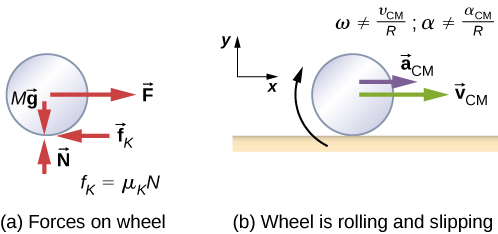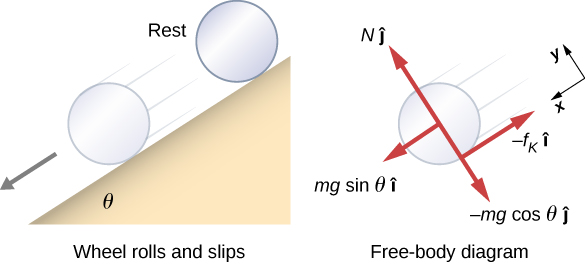11.1 Rolling motion  (Page 3/6)

 Page 3 / 6

Significance

1. The linear acceleration is linearly proportional to $\text{sin}\phantom{\rule{0.2em}{0ex}}\theta .$ Thus, the greater the angle of the incline, the greater the linear acceleration, as would be expected. The angular acceleration, however, is linearly proportional to $\text{sin}\phantom{\rule{0.2em}{0ex}}\theta$ and inversely proportional to the radius of the cylinder. Thus, the larger the radius, the smaller the angular acceleration.
2. For no slipping to occur, the coefficient of static friction must be greater than or equal to $\left(1\text{/}3\right)\text{tan}\phantom{\rule{0.2em}{0ex}}\theta$ . Thus, the greater the angle of incline, the greater the coefficient of static friction must be to prevent the cylinder from slipping.

Check Your Understanding A hollow cylinder is on an incline at an angle of $60\text{°}.$ The coefficient of static friction on the surface is ${\mu }_{S}=0.6$ . (a) Does the cylinder roll without slipping? (b) Will a solid cylinder roll without slipping?

a. ${\mu }_{\text{S}}\ge \frac{\text{tan}\phantom{\rule{0.2em}{0ex}}\theta }{1+\left(m{r}^{2}\text{/}{I}_{\text{CM}}\right)}$ ; inserting the angle and noting that for a hollow cylinder ${I}_{\text{CM}}=m{r}^{2},$ we have ${\mu }_{\text{S}}\ge \frac{\text{tan}\phantom{\rule{0.2em}{0ex}}60\text{°}}{1+\left(m{r}^{2}\text{/}m{r}^{2}\right)}=\frac{1}{2}\text{tan}\phantom{\rule{0.2em}{0ex}}60\text{°}=0.87;$ we are given a value of 0.6 for the coefficient of static friction, which is less than 0.87, so the condition isn’t satisfied and the hollow cylinder will slip; b. The solid cylinder obeys the condition ${\mu }_{\text{S}}\ge \frac{1}{3}\text{tan}\phantom{\rule{0.2em}{0ex}}\theta =\frac{1}{3}\text{tan}\phantom{\rule{0.2em}{0ex}}60\text{°}=0.58.$ The value of 0.6 for ${\mu }_{\text{S}}$ satisfies this condition, so the solid cylinder will not slip.

It is worthwhile to repeat the equation derived in this example for the acceleration of an object rolling without slipping:

${a}_{\text{CM}}=\frac{mg\phantom{\rule{0.2em}{0ex}}\text{sin}\phantom{\rule{0.2em}{0ex}}\theta }{m+\left({I}_{\text{CM}}\text{/}{r}^{2}\right)}.$

This is a very useful equation for solving problems involving rolling without slipping. Note that the acceleration is less than that for an object sliding down a frictionless plane with no rotation. The acceleration will also be different for two rotating cylinders with different rotational inertias.

Rolling motion with slipping

In the case of rolling motion with slipping, we must use the coefficient of kinetic friction, which gives rise to the kinetic friction force since static friction is not present. The situation is shown in [link] . In the case of slipping, ${v}_{\text{CM}}-R\omega \ne 0$ , because point P on the wheel is not at rest on the surface, and ${v}_{P}\ne 0$ . Thus, $\omega \ne \frac{{v}_{\text{CM}}}{R},\alpha \ne \frac{{a}_{\text{CM}}}{R}$ .(a) Kinetic friction arises between the wheel and the surface because the wheel is slipping. (b) The simple relationships between the linear and angular variables are no longer valid.

Rolling down an inclined plane with slipping

A solid cylinder rolls down an inclined plane from rest and undergoes slipping ( [link] ). It has mass m and radius r . (a) What is its linear acceleration? (b) What is its angular acceleration about an axis through the center of mass?

Strategy

Draw a sketch and free-body diagram showing the forces involved. The free-body diagram is similar to the no-slipping case except for the friction force, which is kinetic instead of static. Use Newton’s second law to solve for the acceleration in the x -direction. Use Newton’s second law of rotation to solve for the angular acceleration.

SolutionA solid cylinder rolls down an inclined plane from rest and undergoes slipping. The coordinate system has x in the direction down the inclined plane and y upward perpendicular to the plane. The free-body diagram shows the normal force, kinetic friction force, and the components of the weight m g → .

The sum of the forces in the y -direction is zero, so the friction force is now ${f}_{\text{k}}={\mu }_{\text{k}}N={\mu }_{\text{k}}mg\text{cos}\phantom{\rule{0.2em}{0ex}}\theta .$

Newton’s second law in the x -direction becomes

$\sum {F}_{x}=m{a}_{x},$
$mg\phantom{\rule{0.2em}{0ex}}\text{sin}\phantom{\rule{0.2em}{0ex}}\theta -{\mu }_{\text{k}}mg\phantom{\rule{0.2em}{0ex}}\text{cos}\phantom{\rule{0.2em}{0ex}}\theta =m{\left({a}_{\text{CM}}\right)}_{x},$

or

${\left({a}_{\text{CM}}\right)}_{x}=g\left(\text{sin}\phantom{\rule{0.2em}{0ex}}\theta -{\mu }_{\text{K}}\phantom{\rule{0.2em}{0ex}}\text{cos}\phantom{\rule{0.2em}{0ex}}\theta \right).$

The friction force provides the only torque about the axis through the center of mass, so Newton’s second law of rotation becomes

$\sum {\tau }_{\text{CM}}={I}_{\text{CM}}\alpha ,$
${f}_{\text{k}}r={I}_{\text{CM}}\alpha =\frac{1}{2}m{r}^{2}\alpha .$

Solving for $\alpha$ , we have

$\alpha =\frac{2{f}_{\text{k}}}{mr}=\frac{2{\mu }_{\text{k}}g\phantom{\rule{0.2em}{0ex}}\text{cos}\phantom{\rule{0.2em}{0ex}}\theta }{r}.$

Significance

We write the linear and angular accelerations in terms of the coefficient of kinetic friction. The linear acceleration is the same as that found for an object sliding down an inclined plane with kinetic friction. The angular acceleration about the axis of rotation is linearly proportional to the normal force, which depends on the cosine of the angle of inclination. As $\theta \to 90\text{°}$ , this force goes to zero, and, thus, the angular acceleration goes to zero.

what is the value of x 6yx7y
what is the formula for frictional force
I believe, correct me if I am wrong, but Ffr=Fn*mu
Grant
frictional force ,mathematically Fforce (Ffr) =K∆R where by K stands for coefficient of friction ,R stands for normal force/reaction NB: R = mass of a body ( m) x Acc.due gravity (g) The formula will hold the meaning if and only if the body is relatively moving with zero angle (∅ = 0°C)
Boay
What is concept associated with linear motion
what causes friction?
Elijah
uneven surfaces cause friction Elijah
Shii
rough surfacea
Grant
what will happen to vapor pressure when you add solute to a solution?
how is freezing point depression different from boiling point elevation?
shane
how is the osmotic pressure affect the blood serum?
shane
what is the example of colligative properties that seen in everyday living?
shane
What is motion
moving place to place
change position with respect to surrounding
to which
to where ?
the phenomenon of an object to changes its position with respect to the reference point with passage of time then it is called as motion
Shubham
it's just a change in position
festus
reference point -it is a fixed point respect to which can say that a object is at rest or motion
Shubham
yes
Shubham
A change in position
Lily
change in position depending on time
bassey
Is there any calculation for line integral in scalar feild?
yes I'm available
Mharsheeraz
what is thrust
when an object is immersed in liquid, it experiences an upward force which is called as upthrust.
Phanindra
@Phanindra Thapa No, that is buoyancy that you're talking about...
Shii
thrust is simply a push
Shii
it is a force that is exerted by liquid.
Phanindra
what is the difference between upthrust and buoyancy?
misbah
The force exerted by a liquid is called buoyancy. not thrust. there are many different types of thrust and I think you should Google it instead of asking here.
Sharath
hey Kumar, don't discourage somebody like that. I think this conversation is all about discussion...remember that the more we discuss the more we know...
festus
thrust is an upward force acting on an object immersed in a liquid.
festus
uptrust and buoyancy are the same
akanbi
the question isn't asking about up thrust. he simply asked what is thrust
Shii
a Thrust is simply a push
Shii
the perpendicular force applied on the body
Shubham
thrust is a force of depression while
bassey
what is friction?
MFON
while upthrust is a force that act on a body when it is fully or partially submerged in a liquid
bassey
mathematically upthrust (u) = Real weight (wr) - Apparent weight (wa) u = wr- wa.
Boay
friction is a force which opposes relative motion.
Boay
how did astromers neasure the mass of earth and sun
wats the simplest and shortest formula to calc. for order of magnitude
papillas
Distinguish between steamline and turbulent flow with at least one example of each
what is newtons first law
It state that an object in rest will continue to remain in rest or an object in motion will continue to remain in motion except resultant(unbalanced force) force act on it
Gerald
Thanks Gerald Fokumla
Theodore
Gerald
it states that a body remains in its state of rest or uniform motion unless acted upon by resultant external force.
festus
it that a body continues to be in a state of rest or in straight line in a motion unless there is an external force acting on it
Usman
state's that a body will continue to maintain it present state of or of uniform unless it's being called upon by an external force
bassey
derive the relation above
formula for find angular velocity
w=v^2/r
Eric
v=wr>2
bassey
Why satellites don't fall on earth? Reason?
because space doesn't have gravity
Evelyn
satellites technically fall to earth but they travel parallel to earth so fast that they orbit instead if falling(plus the gravity is also weaker in the orbit). its a circular motion where the centripetal force is the weight due to gravity
Kameyama
Exactly everyone what is gravity?
the force that attrats a body towards the center of earth,or towards any other physical body having mass
hina
That force which attracts or pulls two objects to each other. A body having mass has gravitational pull. If the object is bigger in mass then it's gravitational pull would be stronger.For Example earth have gravitational pull on other objects that is why we are pulled by earth.
Abdur
Gravity is the force that act on a on body to the center of the earth.
Aguenim
gravity is a force of Attraction
bassey
Qn1(a) Why during the day sky seen blue colour? (b)why during the sunset its seen reddish colour ?
Boay
How the atmosphere reacts with the light from the sun
Grant
what are the application of 2nd law
It's applicable when determining the amount of force needed to make a body to move or to stop a moving body
festusBy Mistry Bhavesh